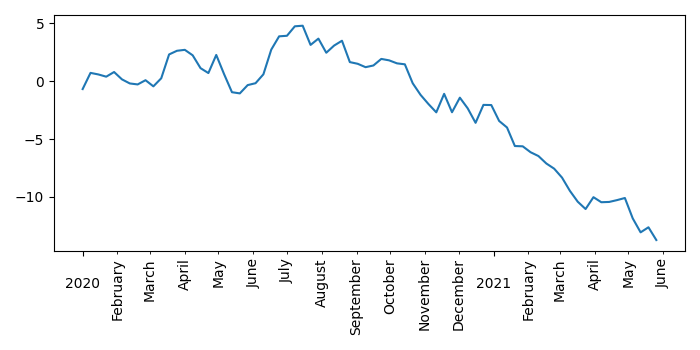# Matplotlib â€“ Date manipulation to show the year tick every 12 months

To make matplotlib date manipulation so that the year tick shows up every 12 months, we can take the following steps −

• Set the figure size and adjust the padding between and around the subplots.
• Create d, y, s, years, months, monthsFmt and yearsFmt using Pandas, Numpy and matplotlib dates.
• Use "%B" in DateFormatter to show full month names.
• Ue "%Y" in DateFormatter to show years.
• Create a new figure or activate an existing figure.
• Add an 'ax' to the figure as part of a subplot arrangement.
• Plot "dts" and "s" data points using plot() method.
• Set minor or major axes locator and formatter. Set minor_locator as months so that the year tick will be displayed every 12 months.
• To display the figure, use show() method.

## Example

import numpy as np
from matplotlib import pyplot as plt, dates as mdates
import pandas as pd

plt.rcParams["figure.figsize"] = [7.00, 3.50]
plt.rcParams["figure.autolayout"] = True

d = pd.date_range("2020-01-01", "2021-06-01", freq="7D")
y = np.cumsum(np.random.normal(size=len(d)))
s = pd.Series(y, index=d)
years = mdates.YearLocator()
months = mdates.MonthLocator()
monthsFmt = mdates.DateFormatter('%B')
yearsFmt = mdates.DateFormatter('\n%Y')

dts = s.index.to_pydatetime()
fig = plt.figure()

ax.plot(dts, s)
ax.xaxis.set_minor_locator(months)
ax.xaxis.set_minor_formatter(monthsFmt)
plt.setp(ax.xaxis.get_minorticklabels(), rotation=90)

ax.xaxis.set_major_locator(years)
ax.xaxis.set_major_formatter(yearsFmt)

plt.show()

## Output

It will produce the following outputUpdated on: 21-Sep-2021

2K+ Views